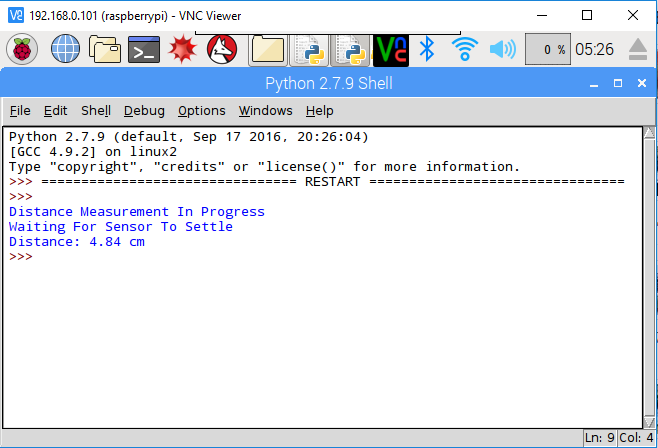# Ultrasonic Distance Sensor in Raspberry Pi

Learn how to use Ultrasonic Distance Sensor in Raspberry Pi. Also, learn how to measure distance using HC-SR04 Ultrasonic Sensor in Raspberry Pi. We will be using Raspberry Pi 3 and HC-SR04 as Ultrasonic Sensor. So, let’s start.

First, let’s start with the basics of the Ultrasonic Distance Sensor HC-SR04.

Operating Voltage: 5V
Current: 15mA
Frequency: 40Hz
Range: 2cm to 400cm
Effectual Angle: 15 degree
Trigger Input Signal: 10uS TTL pulse
Accuracy: ~0.3 cm

## Step 2: Circuit Time

Make the circuit as per the given diagram. There are four pins available in Ultrasonic Distance Sensor. Left-sided pin VCC is connected to 5V, Trigger pin is connected to GPIO 4, Echo pin connected to GPIO 17 and GND pin is connected to Raspberry Pi Ground pin. Connect one end of the 330Ω resistor to the Echo pin. Then, connect a jumper wire from GPIO 17 to another end of the 330Ω resistor. Now, connect one end of the 470Ω resistor to ground and another end at a point where jumper wire from GPIO 17 is connected to the 330Ω resistor.

## Step 3: Code Time

This is code for Ultrasonic Sensor in Raspberry Pi. First import GPIO and time library. Then, declare two pins GPIO 4 for TRIG and GPIO 17 for ECHO. Set the TRIG pin as an output pin and ECHO pin in the Input pin. Then, initialize the sensor by setting the TRIG pin low for 2  seconds. After initializing, it will measure the total duration of a pulse. Now, we multiply the total duration with the speed of sound at sea level. This will give us the distance in centimeter and we have also rounded the result up to two decimal places.

## Step 4: Run code to Raspberry Pi

Run the Python program in your Raspberry Pi after setting components as per the circuit diagram. The Ultrasonic Distance Sensor will give time duration of the pulse to Raspberry Pi and Raspberry Pi will convert the time to distance in centimeter. The screenshot of the output is given below.For running python code in Raspberry Pi, visit: raspberrypi.org/documentation/usage/python/